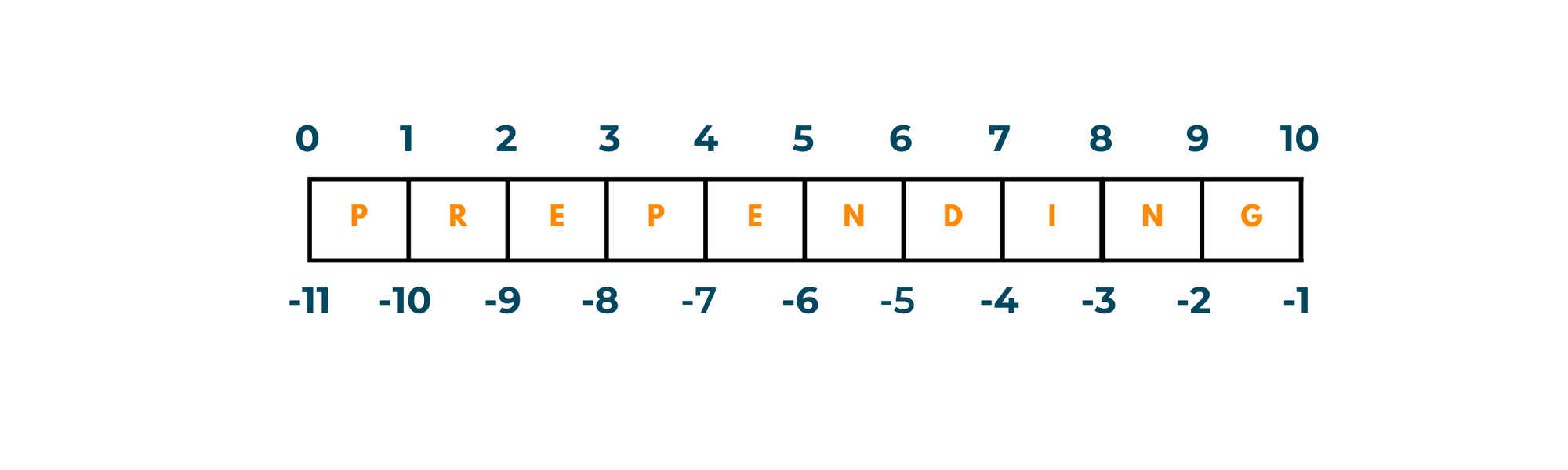# Prepend a List in Python: 4 Best Methods (with code)

Every python programmer should know all the basics of lists, and one of the most important tasks to learn is to add items to them at specified positions. The order of items in a list is significant and sometimes, you might want to add new items to the beginning. In this article, we will learn how to prepend a list in python with code. We will examine several different approaches to it.

But first, let's revisit some important concepts of python lists.

## What is list and prepending a list mean?

A list in Python is a collection of ordered items that can be changed, meaning it is mutable. Items in a list can be of any data type, including numbers, strings, or other lists.

The item is identified by its index which represents its position in the list. Python lists are zero-indexed. It means that the first element in a list has an index of 0, the second element has an index of 1, and so on. There is also negative indexing from the end of the sequence, which starts from -1.The process of adding an item to the front of a list is referred to as prepending a list in python. A new item is inserted at the top of the list, with all other items shifting to the right to make room for the new item.

## How to prepend to a list in python?

There are various methods to prepend a list in python. We will discuss 4 such ways in detail.

### 1) insert() method

The insert() method in Python is a built-in function that allows you to insert an item into a list at a given position. You can prepend a list by inserting an item in the first position of index 0.

The insert() method has the following syntax:

```list.insert(index, item)
```

where the list is the list you want to prepend, the index is the position you want to insert the item, and the item is the item you want to insert. To prepend an item, we need to set the index to 0. Here is an example:

```numbers = [1, 2, 3, 4, 5]

numbers.insert(0, 0)

print(numbers)
```

Output:

``` [0, 1, 2, 3, 4, 5]
```

However, keep in mind that inserting an element into a list can be an expensive operation, especially if the list is very large since it requires shifting all the elements after the insertion point to make room for the new element.

### 2) The '+' operator

The '+' operator is another way to prepend a list. The '+' operator joins two lists together. To prepend a list, make a new list with the item to be prepended and concatenate it with the original list.

The following is the syntax:

```new_list = [item] + list
```

where the item is the item you want to prepend and the list is the original list. For example:

```numbers = [1, 2, 3, 4, 5]

new_list =  + numbers

print(new_list)
```

Output:

```[0, 1, 2, 3, 4, 5]
```

### 3) The '*' operator

In Python, the '*' operator can also be used to prepend a list by making a new list with the item you want to prepend and multiplying it by the number of times you want it to be repeated.

As an example:

```numbers = [1, 2, 3, 4, 5]

new_list =  * (len(numbers) + 1)

new_list[1:] = numbers

print(new_list)
```

Output:

```[0, 1, 2, 3, 4, 5]
```

### 4) Using slicing

Slicing in python is used for extracting a portion of a list. To prepend a list, make a new list with the item you want to prepend and then combine it with the original list using slicing.

The following is the syntax:

```new_list = [item] + list[:]
```

where the item is the item you want to prepend and the list is the original list. For example:

```numbers = [1, 2, 3, 4, 5]

new_list =  + numbers[:]

print(new_list)
```

Output:

```[0, 1, 2, 3, 4, 5]
```

Each of these methods has advantages and disadvantages, so it is critical to select the one that is best suited to your specific use case. Note that all these methods operate almost at the same speed so code readability is an important factor when deciding which method to use.

## Conclusion

Lists are a frequently used data structure in python. Items can be added to the front or at the end of the list. Adding items to a list, in the beginning, is called pre-pending. Here we learned different ways for how to prepened a list in python.

### FavTutor - 24x7 Live Coding Help from Expert Tutors!